How much do you know about circles?

# Circles

This Math quiz is called 'Circles' and it has been written by teachers to help you if you are studying the subject at high school. Playing educational quizzes is a user-friendly way to learn if you are in the 9th or 10th grade - aged 14 to 16.

It costs only \$12.50 per month to play this quiz and over 3,500 others that help you with your school work. You can subscribe on the page at Join Us

Can you imagine a world without circles? There would be no wheel, no pulleys, no transport, no machinery. And there would be no reason to give the irrational number 3.14159… its special name. Circles are everywhere, and this high school Math quiz will test your knowledge of the circle facts.

So what is a circle? In geometry terms it is defined as the set of points, or a path, such that every point on the path is the same distance away from another point, the center of the circle. Imagine a post in the middle of a big field. A dog is tied to the post, on a lead that is 5 meters long. If the lead is always tight, what shape on the ground will the dog trace out as it runs around? The lead would be the radius of the circle, and the dog’s path is the circumference.

Circles are so special that they have their own names for their different parts. You need to be familiar with them, and know how they are related to each other. And then there is pi… Pi is just a number, and because it isn’t exact, mathematicians decided to give it a name and a symbol to make it easier to work with. If you measured the diameter, d, and the circumference, C, of a number of circular objects, and divided C by d, you should always get an answer just a bit bigger than 3. For any given circle, pi is the ratio of the circumference to the diameter.

Know your way around circles so you don’t end up in a spin!

Question 1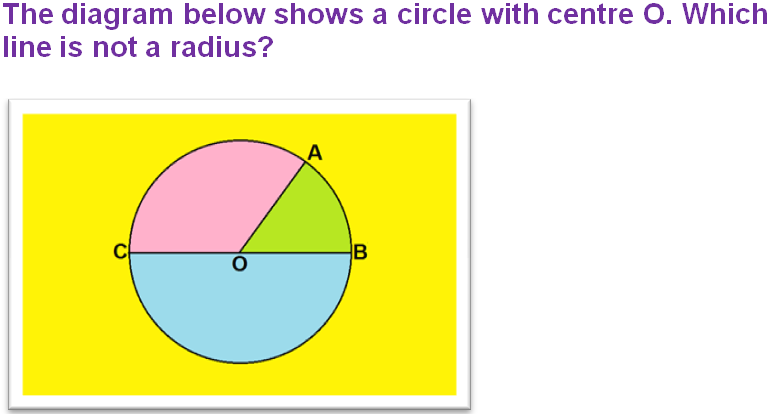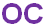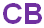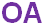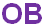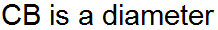Question 2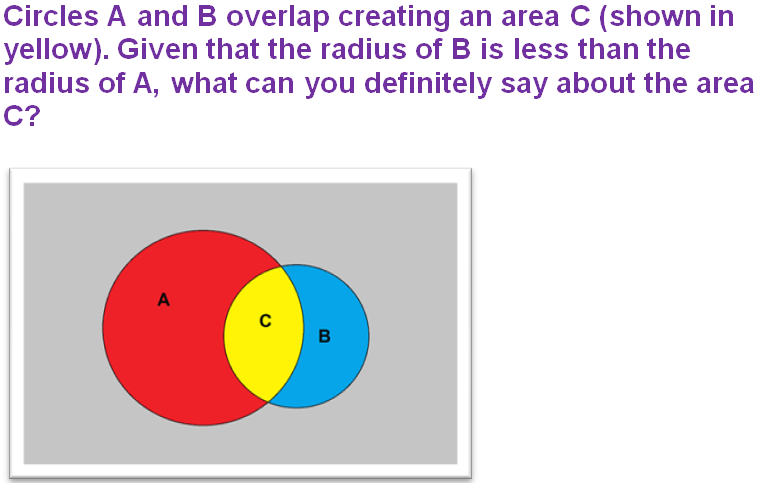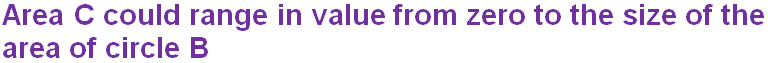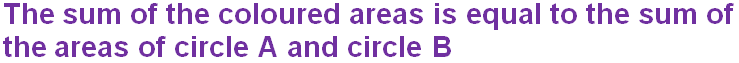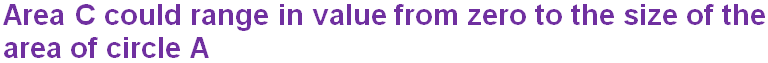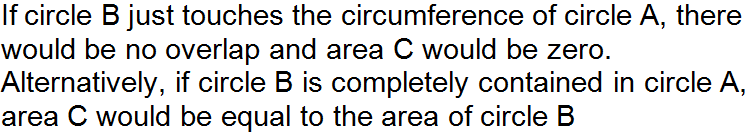Question 3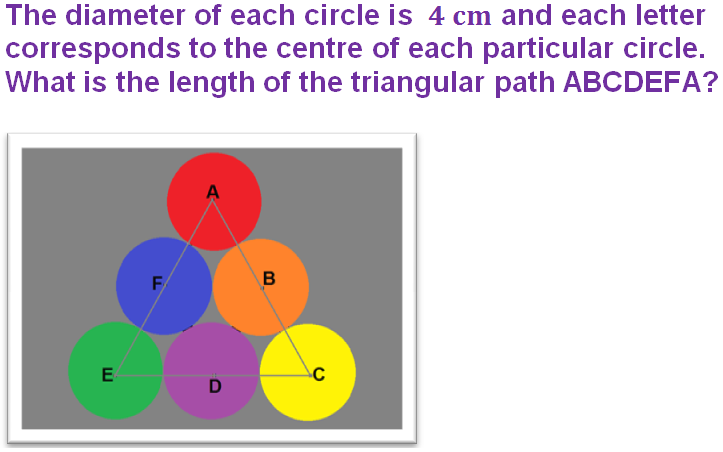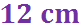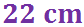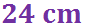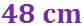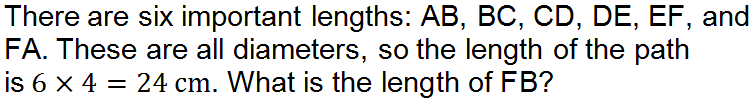Question 4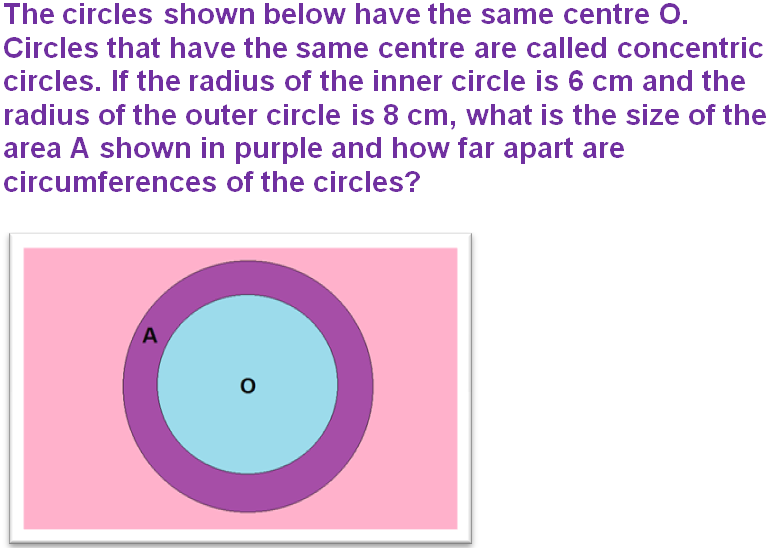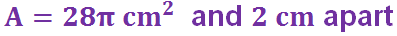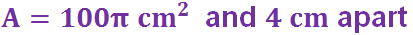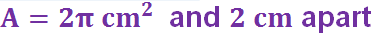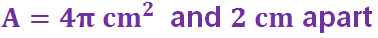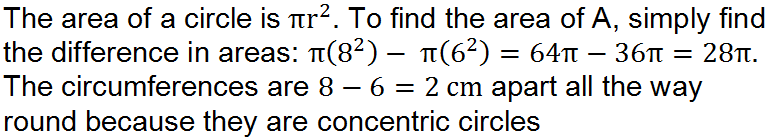Question 5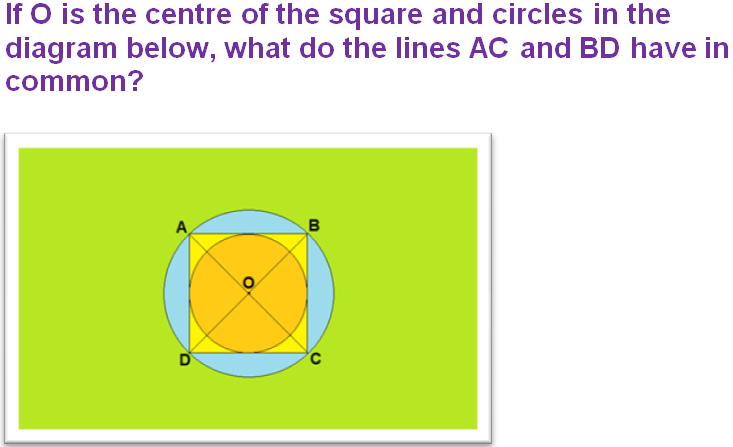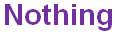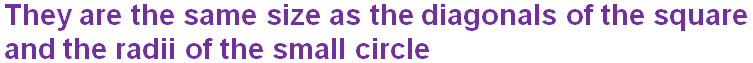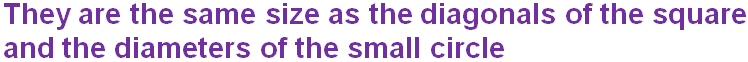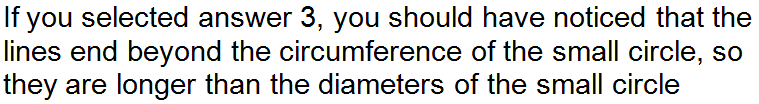Question 6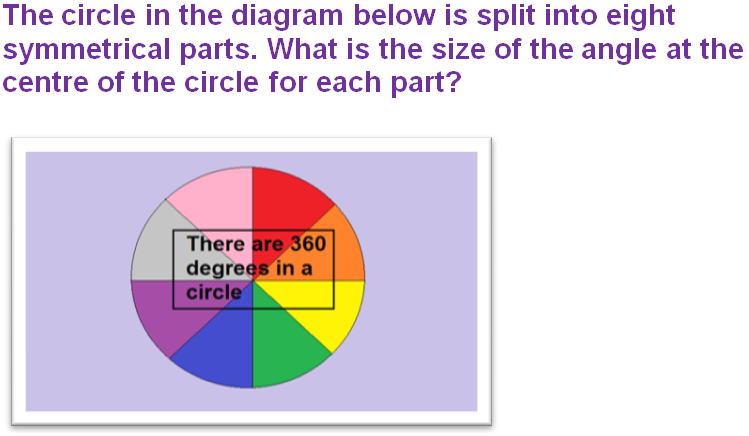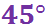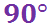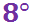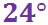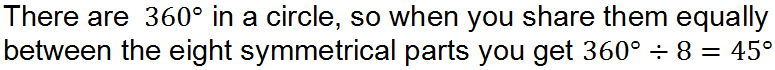Question 7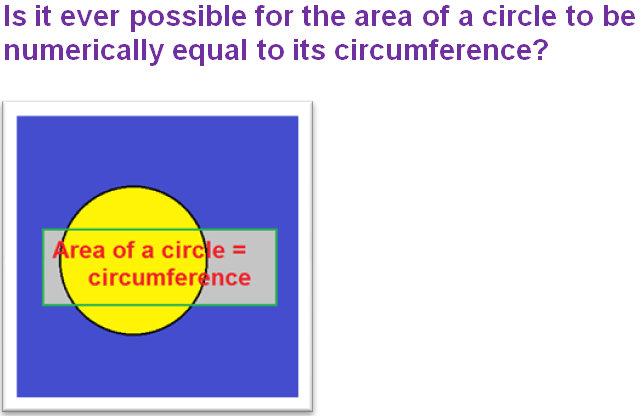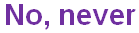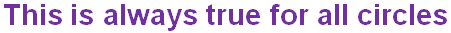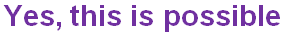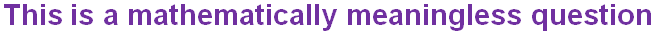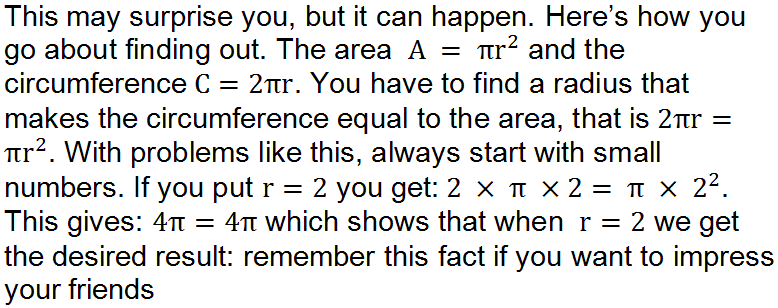Question 8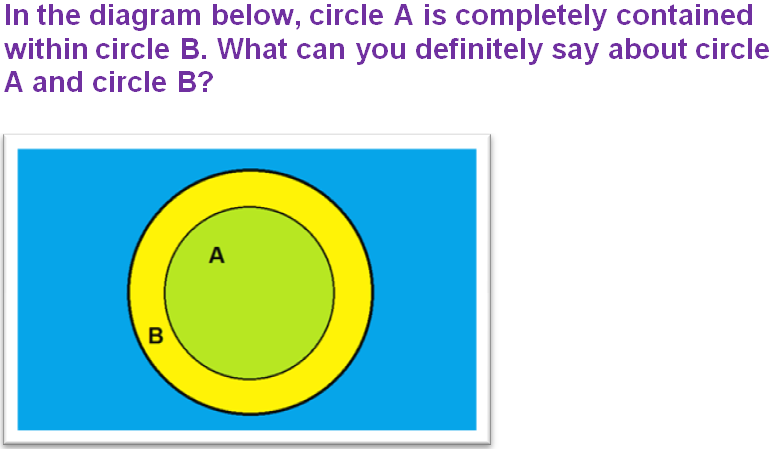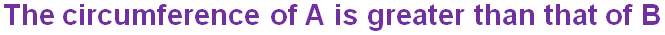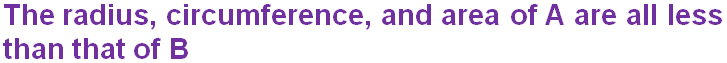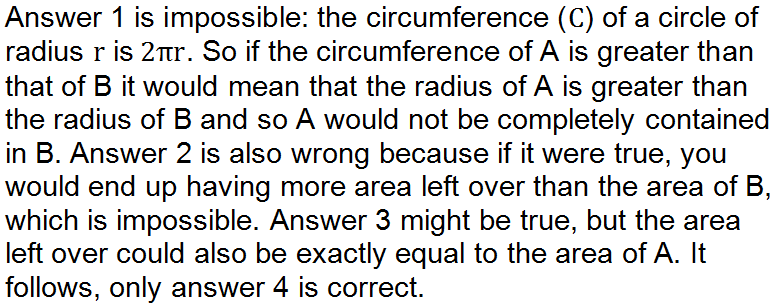Question 9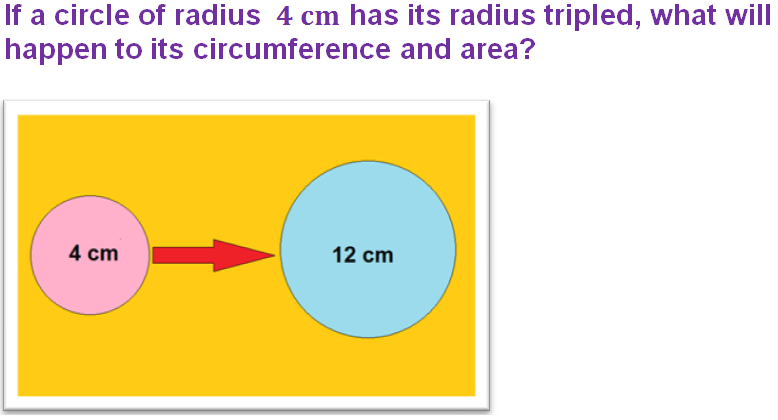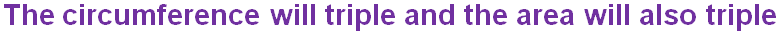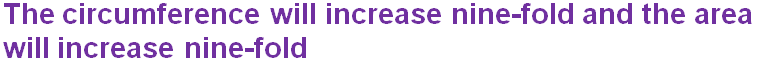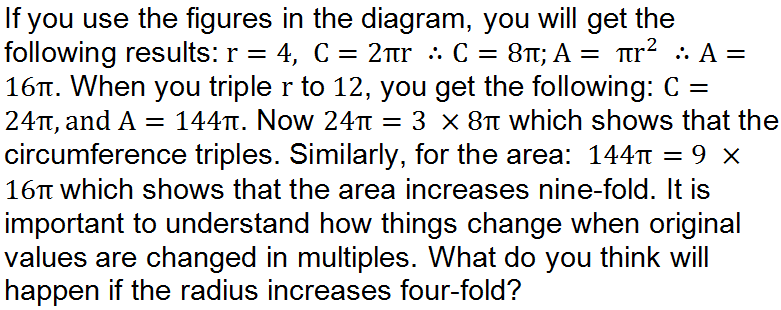Question 10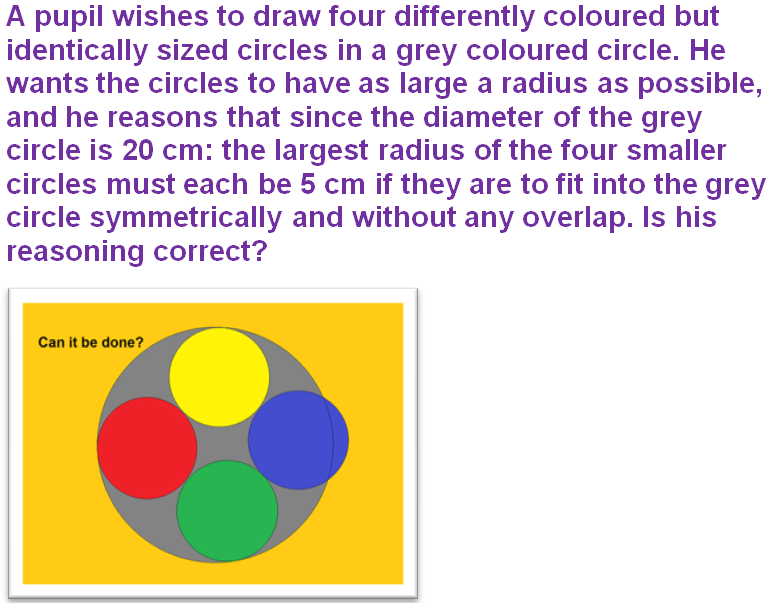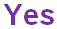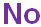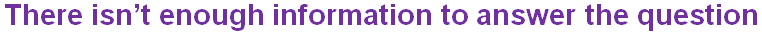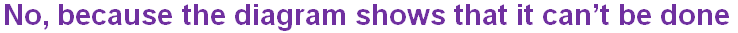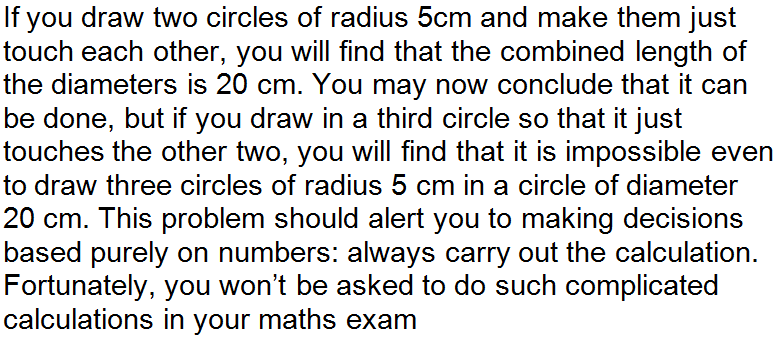Author:  Frank Evans